A Source Book in Greek Science

Contents:
Author: Claudius Ptolemy

Show Summary

PHYSICS

# AN INVESTIGATION OF Refraction

Ptolemy(?), Optics V, pp. 142.1–150.5 (Govi)1

Visual rays may be altered in two ways: (1) by reflection, i.e., the rebound from objects, called mirrors, which do not permit of penetration [by the visual ray], and (2) by bending [i.e., refraction] in the case of media which permit of penetration and have a common designation ["transparent"] for the reason that the visual ray penetrates them.

Now in the preceding books we dealt with mirrors, and explained so far as was possible according to the aforementioned principles of the science of optics, the relation of images and objects, and how the object in each case comes to produce the particular image. It remains for us to consider here the illusions involved when the objects are seen in media through which sight penetrates.

Now it has been shown in what precedes: (1) that this type of bending of a visual ray does not take place in all liquids and rare media, but that a definite amount of bending takes place only in the case of those media that have some likeness to the medium from which the visual ray originates, so that penetration may take place,1 (2) that a visual ray proceeds along a straight line and may be naturally bent only at a surface which forms a boundary between two media of different densities, (3) that the bending takes place not only in the passage from rarer and finer media to denser (as is the case in reflection) but also in the passage from a denser medium to a rarer, and (4) that this type of bending does not take place at equal angles but that the angles, as measured from the perpendicular, have a definite quantitative relationship.2

We must now consider the effect of particular increments in the angles under discussion. Let us, however, first point out what refraction has in common with reflection, namely, that in either case the image appears to be on the prolongation of the straight line forming the initial portion of that ray which passes from the eye and is reflected or refracted to the object.1 That is, the image appears at the intersection of this ray (produced), which passes from the eye to the reflecting or refracting surface, and the perpendicular from the object to the same surface. It follows, therefore, in the case of refraction, just as in the case of reflection, that the plane passing through this altered ray is perpendicular to the surface at which the alteration takes place.2

From this basis follow conclusions relevant to the nature of perception and involving a quantitative relation3 as we have shown in the passage dealing with the principles of mirrors.

This is quite clear and obvious4 and we may understand it immediately with the help of a coin placed in a vessel called a baptistir. For suppose that the position of the eye is such that the visual ray emanating from it and just passing over the edge of the vessel reaches a point higher than the coin. Then, allowing the coin to remain in its position, pour water gently into the vessel until the ray which just passes over the edge is bent downward and falls upon the coin. The result is that objects not previously seen are then seen along the straight line passing from the eye to a point above the true position of the object. Now the observer will not suppose that the visual ray has been bent toward the objects but that the objects are themselves afloat and are raised toward the ray. The objects, therefore, will appear on the perpendicular drawn from them to the surface of the water, in accordance with the principles set forth above.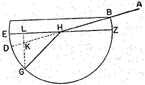Thus [see fig.] if the eye be placed at point A . . . and the coin at point G in the lower part of the vessel, the coin will not be seen so long as the vessel is empty. The reason is that the portion of the vessel just below point B blocks the visual ray which could proceed directly to the coin. But when enough water is poured into the vessel so that it stands at the level ZHE, the ray ABH is bent in the direction of GH, below the prolongation of AH. In that case, the position of the coin will appear to be on the perpendicular from G to EH, that is on LKG which meets AHD at K. The apparent position of the coin, then, will be on the straight line proceeding from the eye and produced so that it passes through K, which is above the line that the visual ray actually takes and nearer to the surface of the water. The coin will, therefore, be seen at point K.

The amount of refraction which takes place in water and which may be observed is determined by an experiment like that which we performed, with the aid of a copper disk, in examining the laws of mirrors.1

On this disk draw a circle [see fig.] ABGD with center at E and two diameters AEG and BED intersecting at right angles. Divide each quadrant into ninety equal parts and place over the center a very small colored marker. Then set the disk upright in a small basin and pour into the basin clear water in moderate amount so that the view is not obstructed. Let the surface of the disk, standing perpendicular to the surface of the water, be bisected by the latter, half the circle, and only half, that is, BGD, being entirely below the water. Let diameter AEG be perpendicular to the surface of the water.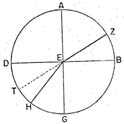Now take a measured arc, say AZ, from point A, in one of the two quadrants of the disk which are above the water level. Place over Z a small colored marker. With one eye take sightings until the markers at Z and at E both appear on a straight line proceeding from the eye. At the same time move a small, thin rod along the arc, GD, of the opposite quadrant, which is under the water, until the extremity of the rod appears at that point of the arc which is on a prolongation of the line joining the points Z and E.

Now if we measure the arc between point G and the point H, at which the rod appears on the aforesaid line, we shall find that this arc, GH, will always be smaller than arc AZ. Furthermore, when we draw ZE and EH, angle AEZ will always be greater than angle GEH. But this is possible only if there is a bending, that is, if ray ZE is bent toward H, according to the amount by which one of the opposite angles exceeds the other.2

If, now, we place the eye along the perpendicular AE the visual ray will not be bent but wilt fall upon G, opposite A and in the same straight line as AE.

In all other positions, however, as arc AZ is increased, arc GH is also increased, but the amount of the bending of the ray will also be progressively greater.1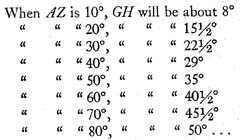2

This is the method by which we have discovered the amount of refraction in the case of water. We have not found any perceptible difference in this respect between waters of different densities.

Now if we make our observation from the relatively dense natural water to the rarer medium, there will be considerable difference in the amount of refraction, corresponding to various increments in the angle of incidence, in the passage of the ray from the denser medium, water, to the rarer. But since it is impossible for us to determine, by an experiment such as that just now described, the amount of refraction which takes place when a visual ray proceeds from a denser to a rarer medium, we have applied the following method of measuring the angles.

Construct a semicylinder of pure glass similar to half the circular disk. Let the base of this semicylinder take the position TKL [see fig.] and let its diameter be smaller than that of the aforesaid metal disk. Fit the base of the semicylinder to the disk so that the whole base is fastened to the disk, the common center is at E, the diameter TL lies along diameter BD, and AE is perpendicular to the plane side of the glass surface. Hence all lines drawn from E to arcs BGD and TKL will be perpendicular thereto.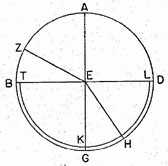Now we arrange this experiment as we did the preceding experiment, placing a small marker on the glass just over E, the midpoint of the straight edge of the base of the semicylinder. We then look, with one eye, along line AE toward the edge of the glass, and keep moving an object along that part of the circumference opposite that from which we are observing, until it appears before our eye. Now this object will be found to be over point G, for AEG is perpendicular both to TEL and TKL.

Again, if we shift our viewpoint to a point opposite A and look in the direction of GE, an object moved over the opposite circumference of the disk will come into view on a prolongation of GE, that is, above EA. For the same reason as before, there will be no bending of the visual ray in its passage from glass to air.1

But now take a certain arc measured from A, say arc AZ, draw ZE, coloring it black, and direct the vision along this line until an object moved back and forth behind the glass is seen in the direction of that line. If we place a marker at the point, H, reached when EH appears collinear with the black line ZE, we shall again in this case find that angle AEZ is larger than angle GEH. Moreover we shall find the excess of the one angle over the other greater than in the case of water, for given angles of incidence.2

And again, when the eye is at point H on the other side of E and looks from H in the direction of HE, both points will appear to be on precisely the same line as in the preceding case. But since there was a bending of the ray at point E, it follows that whether the ray proceeded from the air to the glass, as did ZE, and was bent along EH, or proceeded from the glass to the air, as did HE, and was bent along EZ, in either case there was a bending in the direction of T. And since the perpendiculars which are drawn from E to arc TKL are all similar, they are not bent, whether the rays which they represent are considered as beginning or as ending at E.3

Now if in this case, too, we wish to find the amount of the refraction in each position, we place the eye successively in each of the positions taken in the former experiment [where the visual ray passed from air to water] and thus we vary the angle made on the disk4 between the perpendicular AE and the visual ray EZ. The results are as follows: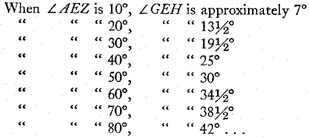5

But the amount of refraction will be less when the glass is placed next to water, since the difference between angles of incidence and refraction in the passage of a visual ray from one of these bodies to the other is not large. For the difference in density between water and glass is less than that between air and water or between air and glass. But we are able again in this case to determine the amount of refraction, as we shall now explain.

Attach a semicylinder of glass [see fig.] to the bronze disk and adjust it so that the center of the straight edge is the same as that of the disk. Again color point E, and set up the bronze disk in a basin so that the disk is at right angles to the surface of the water and half under the water. Place the curved side of the glass, TKL, above, and pour into the basin an amount of water so that edge TEL of the cylinder will be just above the surface of the water.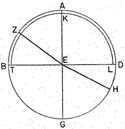Now take arc GH in the less dense medium, that is, in the water, containing, say, 10°. Mark H with a small colored marker, and sight it with one eye until an object Z, which is being moved over arc AB, is seen along the line joining H with the marked point E. When this has been done, draw the two lines EH and EZ.

If, then, we wish to measure on arc AB the angle subtended in the denser medium, that is, in the glass, as the angle in the water measured from the perpendicular, that is, angle GEH, varies, we shall find the following results: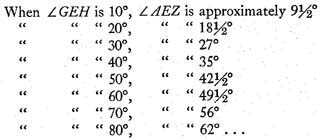1

# SUMMARY OF THE LAWS OF Refraction

Ptolemy(?), Optics V, pp. 154.1–156.6 (Govi)

Now it is possible, on the basis of our investigations, to draw general conclusions about this type of refraction. Thus, near the point at which the visual ray bends and at which a perpendicular drawn from any external point reaches the surface that bounds the two aforesaid dissimilar bodies,1 objects in the denser medium appear larger than they do in the rarer medium (the same position in each medium being preserved). The visual ray passes in this case from the rarer to the denser medium. The opposite will be the case when the passage of the visual ray is from the denser to the rarer medium.2

Our proposition is that the amount of the refraction is the same in each of the two types of passage but that the two refractions differ in type. For in its passage from a rarer to a denser medium the ray inclines toward the perpendicular, whereas in its passage from a denser to a rarer medium it inclines away from the perpendicular,3

For consider a plate such as we have previously described, with diameter BD [see fig.] lying on the surface which divides two dissimilar media.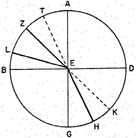Draw the perpendicular AEG. Let a ray inclined to the perpendicular, for example EH, make ∠ GEH with the perpendicular. Now the position of the refracted ray remains exactly the same when the visual ray passes through point E4 and the position of the eye is at point Z. For the line beyond the point of refraction, that is EK inclines, in its course, toward the perpendicular,5 whereas the visible object appears to be along the straight line (EK). Again, if the eye is at point H, and EZ is in the rarer medium (BAD), line ET (beyond the point of refraction) will incline away from perpendicular AE,6 an action exactly the opposite of that in the former case. That is, the ray is further from the perpendicular than if it were to proceed in a straight line.

Again when the media differ considerably (in density), so do the angles (of incidence and refraction), and the difference in the angles becomes greater as the density of (the denser) one of the media is increased. For if we assume that semicircle BAD is in a rarer and BGD in a denser medium, and take angle AEZ as it is [see fig.], then, if the medium of section

BGD is made denser, the excess of ∠ AEZ over ∠ GEH will vary with the excess of the density of the new medium over the old.1 For example, when ∠ AEZ in air is a third of a right angle, ∠ GEH will, in water, be about a fourth part of a right angle, and, in glass a fifth part of a right angle plus a sixtieth thereof, approximately. In this latter case the amount of the refraction and the excess of the angle of incidence over the angle of refraction will, as the former approaches 90°, be greater, since glass is a denser substance than water.

In the same way, if we take the path of one of the refracted visual rays, say LEK, other than that of perpendicular AE,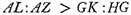and, alternando,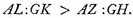Again, separando,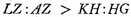and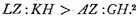2

Now it is possible for us to understand these various points from a quantitative study of the refractions we have investigated, if we assume certain numbers,3 and, with their help take up the several changes indicated on the basis of such initial assumptions, as we did in the case of the two arcs AZ and AL.

But some one in opposition to this may ask why it is that in the first principles set forth—i.e., about the perpendiculars, and the appearance of the image in the direction of the visual ray—there is a similarity between the type of bending just discussed [i.e., refraction] and reflection, as it takes place in mirrors, but there is no such similarity in the measure of the angles.1 The answer, as well as the necessity that things be so, will be found in what we are to set forth. And from this will be seen something even more remarkable, namely, the operation of nature in conserving the activity of force.2

# ATMOSPHERIC Refraction

Ptolemy(?), Optics V, pp. 151.1–153.20 (Govi)

Again, it is possible for us to see from the phenomena which I am about to discuss that at the boundary between air and aether there is a bending of the visual ray because of a difference between these two bodies. We find that stars which rise and set seem to incline more toward the north when they are near the horizon and are measured by the instrument used for such measurement.3 For the circles, parallel to the equator, described upon these stars when they are rising or setting are nearer the north than the circles described upon them when they are in the middle of the heaven.4 As they draw nearer the horizon they have a greater inclination toward the north. In the case of stars which do not rise and set, their distance from the north pole will be smaller when their position on the meridian is nearer the horizon. For when their position on the meridian is nearer the zenith, the circle parallel to the equator will, at that point, be larger, whereas it is smaller in the other position.5 This is due to the bending of the visual ray at the surface which divides the air from the aether, a spherical surface, of necessity, whose center is the common center of all the elements and of the earth.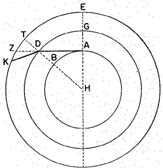Consider, then, in the first place point E [see fig.] as the zenith and the great circles of the various spheres which we have mentioned, circle AB on the surface of the earth, circle GD on the surface dividing air and aether, and EZ which passes through certain stars. Let the center of all the spheres be H. Draw EAH, take point A as the position of the eye, and line ADZ to meet the boundary common to the horizon and to circle GD. Again, let DT be perpendicular to the circle, and consider ADK as a visual ray bent from point D along KD. Suppose there is a star at point K. Since the visual ray is bent at the surface,1 toward a position more remote from point E, the angle KDT (which is in the rarer medium) formed with the line [HDT] perpendicular to the bounding surface from which a reflection would be at equal angles, is greater [than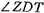]. The stars will therefore be seen from point A2 along line ADZ, and the distance of the star from the zenith will appear less than the true distance. For its distance will appear to be arc EZ instead of arc KE.

The higher, then, the position of the star in the heavens, the smaller will be the difference between its apparent and its real position. If the star is at E there is no bending, since the visual ray from point A to point E is not subject to such bending (refraction), for it is perpendicular to the bounding surface at which any refraction would take place.

These, then, are the preliminary propositions. Let us now consider ABG [see fig.] as the circle of the horizon, and AEZG as that half of the circle of the meridian which is above the earth, E being the zenith and Z the visible pole of the celestial sphere. Let BHD be the portion above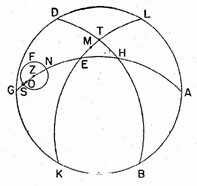earth of the circle parallel to the celestial equator and passing through certain stars. Let there be a star at point T on this circle near the horizon, and let KETL be the visible half of the circle which passes through the zenith and through the star at T. Since, then, when a star is near the horizon it appears nearer the zenith than it really is,3 and its apparent position differs from its true position on the great circle [passing through the zenith and the star in question and] intersecting the horizon, it follows that the apparent position of the star, which is above point T, will be between E and T, let us say at point M. Then the circle parallel to the celestial equator and passing through M will lie further to the north than the circle (parallel to the celestial equator) passing through point T, which, in our part of the inhabited world, lies toward the north. When the star has risen to point H it is in a position where the bending of the visual ray is insufficient to cause a perceptible difference between the apparent and the true position.4

Similarly, take Z as the north celestial pole, and let NSF be the circle parallel to the celestial equator, described by one of the stars which are always above the horizon. When the star is at point S of this circle it will appear nearer point E, the zenith, and will seem to be at about point O. But when the star is at point N there is no difference, or only an imperceptible difference, between the apparent and the real position. And therefore when such a star, in its revolution, is nearer the horizon, its distance from the north pole of the celestial sphere will seem less than the distance from the aforesaid pole when the star in its revolution is nearer the zenith. For arc ZN will be greater than arc OZ. We have therefore seen why stellar phenomena must, because of the bending of visual rays, appear as they do.1. . .

Cf. Ptolemy, Almagest, p. 13.3–9 (Heiberg)

Now the fact that heavenly bodies appear larger when they are near the horizon is due not to their smaller distance from the horizon but to the vaporous moisture surrounding the earth between our eye and these heavenly bodies.2 It is the same as when objects immersed in water appear larger and in fact the more deeply immersed the larger.

Cf. Cleomedes, On the Circular Motion of the Heavenly Bodies II. 1, pp. 122.15–124.8 (Ziegler)

The sun appears larger to us as it rises and sets, but smaller when it is in mid-heaven, for the reason that in viewing it at the horizon we see it through a thicker layer of air and also one that is more humid, for such is the air next to the earth. But when we view it in mid-heaven, we see it through clearer air. Thus in the latter case the ray issuing from the eye toward the sun is not refracted, but in the former case, where the ray is directed toward the horizon when the sun rises or sets, the ray is necessarily refracted when it encounters the thicker and moister air. Thus the sun appears larger to us, just as the appearance of objects in water is altered because they are not seen in a straight line.3 Such phenomena are in every ease to be considered as due to disturbances of our vision and not connected with the visible objects themselves. It is also said that where it is possible to view the sun from deep wells, its appearance is much larger since it is seen through the humid air of the well. It certainly cannot be said that the sun grows larger when viewed from the bottom of a well and smaller when viewed from the top; but evidently the dimness and dampness of the air in the well cause the sun to appear larger to the observer.

# A "PARADOXICAL" ECLIPSE EXPLAINED BY Refraction

Cleomedes, On the Circular Motion of the Heavenly Bodies II. 6. Translation of T. L. Heath, Greek Astronomy1

These facts having been proved with regard to the moon, the argument establishing that the moon suffers eclipse through falling into the earth’s shadow would seem to be contradicted by the stories told about a class of eclipses seemingly paradoxical. For some say that an eclipse [sometimes] occurs, even when both the luminaries are seen above the horizon.2 This should make it clear that [in that case] the moon does not suffer eclipse through falling into the earth’s shadow, but in some other way, since, if an eclipse occurs when both sun and moon appear above the horizon, the moon cannot suffer eclipse through falling into the earth’s shadow. For the place where the moon is, when both bodies appear above the horizon, is still being lit up by the sun, and the shadow cannot vet be at the place where the moon gives the impression of being eclipsed. Accordingly, if this be the case, we shall be obliged to declare that the cause of the eclipse of the moon is a different one.3. . .

Nevertheless, having regard to the many and infinitely various conditions which naturally arise in the air, it would not be impossible that, when the sun has just set, and is under the horizon, we should receive the impression of its not yet having set, if there were cloud of considerable density at the place of setting and the cloud were illuminated by the sun’s rays and transmitted to us an image of the sun, or if there were "anthelium." Such images are indeed often seen in the air, especially in the neighbourhood of Pontus. The ray, therefore, proceeding from the eye and meeting the air in a moist and damp condition might be bent, and so might catch the sun although just hidden by the horizon. Even in ordinary life we have observed something similar. For, if a gold ring be thrown into a drinking cup or other vessel, then, when the vessel is empty, the object is not visible at a certain suitable distance, since the visual current goes right on in a straight line as it touches the brim of the vessel. But, when the vessel has been filled with water up to the level of the brim, the ring placed in the vessel is now, at the same distance, visible, since the visual current no longer passes straight on past the brim as before, but, as it touches, at the brim, the water which fills the vessel up to the brim, it is thereby bent, and so, passing to the bottom of the vessel, finds the ring there. Something similar, then, might possibly happen in a moist and thoroughly wet condition of the air, namely, that the visual ray should, by being bent, take a direction below the horizon, and there catch the sun just after its setting, and so receive the impression of the sun’s being above the horizon. Perhaps, also, some other cause akin to this might sometimes give us the impression of the two bodies being above the horizon, though the sun had already set. But the observed phenomena make it as clear as day that the moon is not eclipsed otherwise than by falling within the earth’s shadow.

1 This very obscure passage seems to mean that the process of refraction (as opposed to that of reflection) requires two different media, but not so different that one of them does and the other does not allow a visual ray to penetrate.

2 Precisely what is meant is difficult to say because of the obscurity of the text. It is tempting, however, to interpret the passage as referring to a quantitative relation between angle of incidence and angle of refraction (the incident ray passing from the eye, and the angles being measured from the perpendicular upon the surface dividing the media). From the way in which the results of the observations seem to have been corrected, as the tables given on p. 278 indicate, the relation was of the form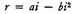(where i is the angle of incidence, r the angle of refraction, and a and b constants depending on the specific media). This does not accord with accurate modern observation as well as does the relation sin i/sin r = constant, but it is more accurate than the relation i/r = constant, which seems to have been widely adopted (see the tables, p. 278). Throughout the discussion it is to be remembered that the ray from the eye is considered the incident ray, in contrast with modern usage, in which the ray from the object is generally so named.

1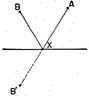That is, if A be the eye, B the object, B′ the image, and X the point where the initial portion of the ray which is reflected to the object impinges on the reflecting or refracting surface, the image appears on a prolongation of AX.

2 What seems to be meant is that the plane containing the visual and refracted rays is perpendicular to the refracting surface, just as, in the case of reflection, the plane containing the visual and reflected rays is perpendicular to the reflecting surface.

3 In the case of reflection, the equality of the angles of incidence and reflection.

4 The reference seems to be to the rule for the location of the image in the case, now, of refraction.

1 See p. 270, above.

2 The amount of bending depends on the law connecting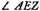and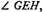angles of incidence and refraction, respectively.

1 That is, GH increases but at a progressively slower rate than AZ.

2 See the comparisons in Table A, From Air to Water, below. Note that r, the angle of refraction, is given by the equation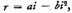where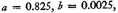and i is the angle of incidence (the incident ray being that which passes from the eye to the refracting surface: modern usage generally calls this the angle of refraction).

1 I.e., there is no refraction when the visual ray is perpendicular to the boundary between the media. Thus there is no refraction in the light from a star at the zenith (see p. 282).

2 I.e., the excess of the angle of incidence over the angle of refraction is greater in the passage of the visual ray from air to glass than for the passage from air to water.

3 The point seems to be that so long as the ray is within the same medium, glass, there is no bending.

4 Possibly, "in the air."

5 See the comparisons in Table B, From Air to Glass. Note that the angle of refraction is given by the equation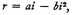where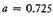and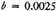.

1 That is,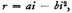where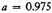and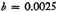(Table C, below).

The following tables will serve to compare the theory of refraction as given in our text with that in which the ratio of the angles of incidence and refraction is a constant, and with the modern theory, in which the ratio of the sines of the angles is a constant. See P. Brunet and A. Mieli, Histoire des sciences: antiquité, pp. 826–827.

It may be noted that the correction of experimental data to correspond to a set formula is discussed by Kepler in connection with Witelo’s (thirteenth century) tables of refraction, which are much the same as those in the present text. Kepler contented himself with the formula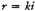for relatively small angles of incidence. The relation between refraction and the velocity of light in different media (which leads to the sine law) was not explicitly stated until the seventeenth century.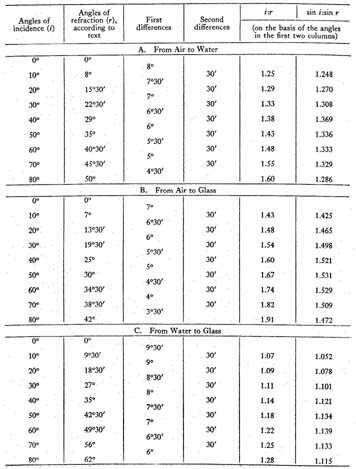1 The boundary between the air and the aether had been referred to, hut the sequel considers any two media of different densities.

2 The point seems to be that if the eye and the object exchange positions the object (now in the rarer medium) appears smaller than it did in the denser medium.

3 Here we have the general statement of the reversibility of refraction. The experimental evidence is set forth in the following paragraphs.

4 I.e., originates further back than E.

5 Obviously what is meant is that the visual ray, which if unbent would lie along EK, is bent toward the perpendicular.

6 I.e., the ray, which if unbent would lie along ET, is bent away from the perpendicular.

1 Neither the method of measuring relative densities nor the precise way in which the angular differences vary with the differences in densities can be gathered from the example that follows, viz., when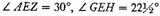in water and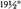in glass (see p. 278, above).

2 If i1 and i2 are angles of incidence (the incident visual ray being that which passes from the eye to the refracting surface) with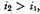and r1 and r2 the corresponding angles of refraction, the preceding paragraph gave the result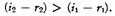The present paragraph gives the results: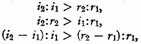and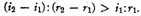But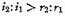(from which the other results follow immediately) is valid only in the case where the visual ray from the eye passes from the less dense to the denser medium (the angle of incidence being the angle made by the line of vision with the perpendicular), as in all the cases given by the author. Since we, however, consider the light as passing from the object to the eye, and take the angle of incidence as that angle which the ray from the object makes with the perpendicular, it follows that, for us,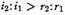only in the passage of light from the less refrangent to the more refrangent medium: otherwise,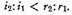3 The point seems to be that the theoretical results set forth above may be better grasped if we assign specific values to i2 and i1, and note the corresponding values of r2 and r1 (from tables such as those on p. 278).

1 The angles of incidence and reflection are equal, but the angles of incidence and refraction are unequal.

2 This statement of a principle of least action or conservation of energy is to be noted, though its application to the problem of refraction is not clearly made by the author in the portion of Book V that is extant.

3 The dioptra or the astrolabe (see p. 139).

4 I.e., the small circle (parallel to the celestial equator) that represents the apparent path of a star in its diurnal rotation appears to have a greater declination when taken at the rising or setting of the star in question than, say, midway between the rising and setting.

5 I.e., the apparent distance from a circumpolar star to the pole is greater at its upper than at its lower transit across the meridian.

1 I.e., the surface marking the boundary between aether and air.

2 The text reads E, which can hardly be right.

3 The text reads "nearer the zenith than to its true position," which is obviously wrong.

4 At H the star is on the meridian, and its angular distance from the zenith is least.

1 The author goes on to indicate the impossibility of measuring astronomical refraction because of the absence of data as to the relative extent of the atmosphere and the aether.

2 The apparent increase of size of sun and moon at the horizon is largely due to an optical illusion, not to refraction (cf. also p. 123).

3 But by a refracted ray.

1 It is not known when Cleomedes lived. Some place him in the first century B.C., others in the first or second A.D. He was a compiler rather than an original scientist, and his work is important for the wealth of historical information that it contains. His treatise On the Circular Motion of the Heavenly Bodies, extant in two books, deals with various astronomical questions and is often controversial, reflecting the Stoic viewpoint against the Epicurean. A passage containing the account of the methods used by Eratosthenes and Posidonius in estimating the size of the earth has been quoted above (p. 149). The present passage discusses possible explanations of a "paradoxical" eclipse of the moon when both sun and moon appear above the horizon. [Edd.]

2 Pliny refers to an instance of such an eclipse (Natural History II. 57) as follows: "And [it was discovered by Hipparchus] why it is that, though after sunrise the eclipsing shadow must be below the earth, it has once happened that the moon was eclipsed in the west while both sun and moon were visible above the horizon."

Such an eclipse was visible in the vicinity of New York City on Nov. 7, 1938. [Edd.]

3 Cleomedes is skeptical about the reported observation, but considers various explanations before turning to the possibility of refraction as an explanation. [Edd.]

Contents:

### Related Resources

Ptolemy

Title: A Source Book in Greek Science

Select an option:

## Email Options

Title: A Source Book in Greek Science

Select an option: